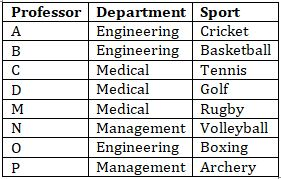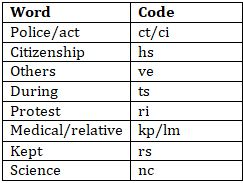Latest Banking jobs   »   Reasoning Ability Quiz For IBPS Clerk...

# Reasoning Ability Quiz For IBPS Clerk Prelims 2021- 7th August

Directions (1-5): Study the following information carefully and answer the questions given below.
Eight professor- A, B, C, D, M, N, O, and P are working in three departments Engineering, Medical, and Management of an Institute with at least two of them work in each department and not more than three persons work in same departments. Each of them likes a different sports Golf, Volleyball, Archery, Boxing, Tennis, Rugby, Basketball and Cricket but not necessarily in the same order. P likes Archery and he works in Management department with only N. B and M do not work in the same department. O likes Boxing and does not work in Medical department. M and C works together but not in engineering department. D works with C in the same department and likes Golf. B likes Basketball and none of his colleagues in the department likes Tennis and Rugby. N likes Volleyball and M does not like Tennis.

Q1. Who among the following works same department as B?
(a) C
(b) D
(c) M
(d) O
(e) P

Q2. Which of the following sport likes by M?
(a) Cricket
(b) Rugby
(d) Volleyball
(e) Tennis

Q3. Four of the following five form a group, which among the following does not belong to that group?
(a) D-Medical
(b) C-Tennis
(c) N-Management
(e) O-Engineering

Q4. Who among the following likes tennis?
(a) C
(b) A
(c) M
(d) O
(e) N

Q5. Which of the following group of persons work in the same department?
(a) N-O
(b) P-C
(c) A-M
(d) O-B
(e) D-A

Directions (6-10): The following questions are based on the five words in each of three letters given below.
TRA PTQ YTV WEN CBN

Q6. If all the letters in each of the words are arranged in alphabetical order within the word, then how many meaningful words will be formed?
(a) One
(b) Two
(c) Three
(d) More than three
(e) None

Q7. If the first letter of each word replace by succeeding letter in English alphabet, then how many words have more than one vowel?
(a) Three
(b) Two
(c) One
(d) More than three
(e) None

Q8. If all the words are arranged in alphabetical order, then how many words remains at the same position?
(a) None
(b) One
(c) Two
(d) Three
(e) More than three

Q9. If first and last letter of each word are interchanged their position, then how many meaningful words are formed?
(a) One
(b) Two
(c) Three
(d) More than three
(e) None

Q10. If all words are arranged in alphabetical order from left after interchanging first and second letter of each word, then which of the following word is 3rd from right?
(a) YTV
(b) TRA
(c) WEN
(d) CBN
(e) None of these

Directions (11-15): Study the following information carefully and answer the questions given below:
In a certain code language,
‘police citizenship act protest’ is written as ‘ri ct ci hs’,
‘during protest medical relative’ is written as ‘kp lm ts ri’,
‘relative medical kept others’ is written as ‘rs kp ve lm’,
‘police act science kept’ is written as ‘nc ct ci rs’.

Q11. Which of the following may be the code for ‘police relative act’?
(a) kp ct rs
(b) ve ci kp
(c) ct ci rs
(d) ct kp ci
(e) Either (c) or (d)

Q12. What is the code for ‘Citizenship’?
(a) ci
(b) ct
(c) ri
(d) hs
(e) None of these

Q13. What is the code for ‘Science’?
(a) rs
(b) ct
(c) nc
(d) ve
(e) Can’t be determined

Q14. What is the code for ‘Police’?
(a) ct
(b) ci
(c) ri
(d) nc
(e) Can’t be determined

Q15. What does ‘lm’ stand for?
(a) Medical
(b) Relative
(c) Kept
(d) Either (a) or (c)
(e) Either (a) or (b)

Solutions

Solutions (1-5):
Sol.S1. Ans.(d)
S2. Ans.(b)
S3. Ans.(d)
S4. Ans.(a)
S5. Ans.(d)

S6.Ans (a)
Sol. ART

S7.Ans (c)

S8.Ans (c)

S9.Ans (b)

S10.Ans (b)

Solutions (11-15):
Sol.S11. Ans. (d)
S12. Ans. (d)
S13. Ans. (c)
S14. Ans. (e)
S15. Ans. (e)